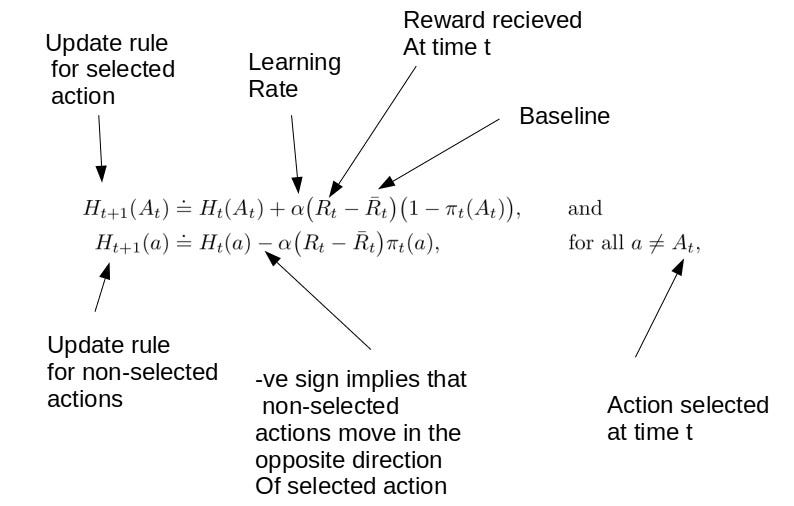# Multi-Armed Bandit — Solution Methods

Original article was published on Artificial Intelligence on Medium# Optimistic Initial Value

Can we promote exploration without using Epsilon-Greedy?

It turns out that we can do this using the concept of Optimistic Initial Value.

## Without Optimistic Initial Value

Let us understand it. Initially we do not know the true reward associated with each action. But we will make estimates as we progress.

At t = 0, estimated_rewards = [0, 0, 0, 0, 0, 0, 0, 0, 0, 0]

Let us assume that we select to pull the first lever and get a reward of 0.2. So average reward for this arm will be (0 + 0.2)/2 = 0.1 .

At t = 1, estimated_rewards = [0.1, 0, 0, 0, 0, 0, 0, 0, 0]

Now because we are following Greedy Action Selection ( not Epsilon), so as we progress in this game we will just keep selecting the first lever. Let the pull of the first lever arm give a reward of 0.18 (remember that the rewards given at runtime are sampled from true rewards). So average reward for this arm will be (0 + 0.2 + 0.18)/3 = 0.126 .

At t =2, estimated_rewards = [0.126, 0, 0, 0, 0, 0, 0, 0, 0]

So we will keep selecting the first arm. Since we are not exploring so there are fair chances that we are missing the optimal return. Can we do better?

## With Optimistic Initial Value

Now suppose we initialize the estimated rewards with a value of +5 each. So initially we have :

At t = 0, estimated_rewards = [5, 5, 5, 5, 5, 5, 5, 5, 5, 5]

Let us again assume that we select to pull the first arm and get a reward of 0.2. So average reward for this arm will be (5 + 0.2)/2 = 2.6.

At t = 1, estimated_rewards = [2.6, 5, 5, 5, 5, 5, 5, 5, 5, 5]

Did you notice that? The estimated average reward from the selected arm is lower than its initial estimates. So our agent will be a bit disappointed and this will motivate the agent to explore other arms. Now the greedy policy has 9 choices ( because the maximum value 5 is associated with 9 other actions). Assuming that ties are broken randomly, a random action from among the remaining 9 will be chosen. Let us suppose the 3rd arm is selected this time. So average reward for this arm will be (5 + 0.2)/2 = 2.6.

At t = 2, estimated_reward = [2.6, 5, 2.6, 5, 5, 5, 5, 5, 5, 5]

Notice that while calculating average we do not divide by the time step. Rather the denominator is the number of times that particular action has been selected in the past.

It is easy to observe that as we continue this way, every arm will be explored at least once. So we observed how Optimistic Initial Value can make a big difference. We can also combine the Optimistic Initial Value technique with epsilon Greedy.

Let’s see some comparison charts:-